# Integrated Time Domain and Frequency Domain Software for 3D RF and Microwave Applications

Modeling and simulation of many RF applications and antennas present a challenge in terms of speed, required memory, and accuracy. For these applications if we move beyond using frequency domain techniques (e.g., boundary element method (BEM)) to time-domain solvers such as finite – difference time-domain (FDTD), huge savings in the running times are possible. The simple example of the conventional rectangular patch antenna, shown in Figure 1, shows the advantages of using our time domain solver. The problem was simulated in eight minutes using a single processor Intel(R) Xeon(R) 2.66 GHz to cover the frequency band 0.5 – 12 GHz, while the same problem should be simulated at 240 frequencies if a frequency domain solver is used. The running time for the simulation at each frequency using INTEGRATED’s frequency domain solver SINGULA (which is based on the Boundary Element Method (BEM)) was two minutes (total running time for the 240 frequencies was 8 hours).

To meet this challenge the company has introduced CHRONOS to its range of modeling and simulation software. The program is based on the Finite Difference Time Domain (FDTD) method, combined with an efficient mesh generator code and special treatments for arbitrary shaped and curved structures. This is ideal for a wide variety of RF and antenna applications and, being based on a direct solution of Maxwell’s equations in the time domain, has the following advantages:

• It provides a high accuracy for a wide variety of RF and antenna problems.
• No storage in time and no matrix inversion are needed.
• It gives the solution for broadband or ultrawideband problems from a single execution of the problem using a short pulse.
• It provides both of the near-field and far-field results from a single run.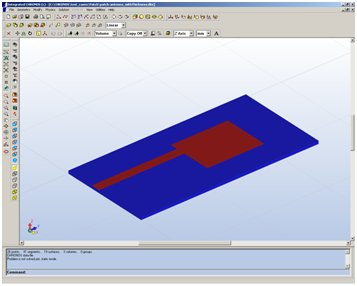Figure 1

Simulation of electrically large structures which have fine features, and general curved structures using the orthogonal FDTD mesh, is always challenging because of the heavy burden they impose on the CPU if the generated mesh is not optimized. For this reason CHRONOS has a smart mesh generator code with the ability to recognize all the details of any structure and decide how to distribute the mesh based on the accuracy requirements of the FDTD algorithm. This gives the user two options, to either generate the mesh distribution manually or automatically using the non-uniform smart mesh generator. The smart mesh generator is used to improve the accuracy of the solution and to reduce the number of cells used in the computational domain, leading to a significant improvement in the used memory and speed. The mesh is generated by placing finer grids in the areas which have fine features and then increasing the cell sizes gradually (to avoid spurious reflections) as the mesh moves away from these areas until the cell size reaches the maximum (usually λ/20) or it reaches near another region that needs fine a grid.

The problem shown in Figure 2 of a metamaterial antenna based on composite right/left hand (CRLH)  is a good example to show the advantage of using the adaptive grid generation. This metamaterial structure includes slots with widths of 0.2 mm and vias of radius 0.15 mm. The frequency of the first negative order resonance (n=-1) occurs at f=2.8 GHz as shown in Figure 2(b). The slot width is 0.001λ (at n=-1) and if a uniform grid is used with cell sizes in the x and y directions ∆x=∆y=0.1 mm , the number of unknown field components in the computational domain is 15.6 million while using adaptive grid reduces the number of unknown field components to 5.7 million.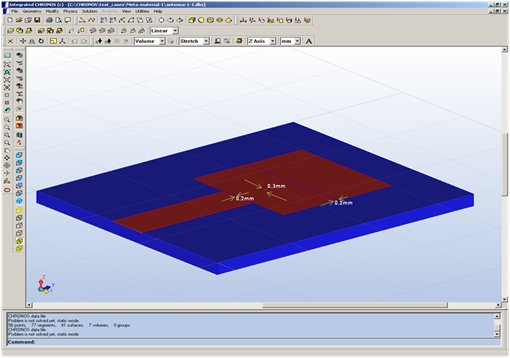Figure 2a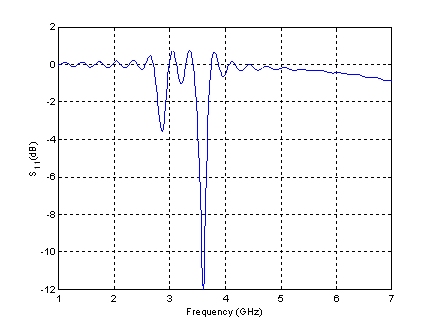Figure 2b

Figure 3 shows the problem of the electromagnetic penetration in a building with dimensions 7m x 10m x 10 m. The building is located at a distance of 3m from an antenna resonating at 1 GHz. The total dimensions of the problem are 7m x 13m x 10m and after adding additional spaces to the absorbing boundary conditions, the problem requires 1.1 billion of unknown field components to be simulated. The running time for this problem was 51 hours and 44 minutes using Intel(R) Xeon(R) E5430@2.66 GHz (2 processors) with 48 GB RAM and 64 bit operating system. Figure 4 shows the distribution of the electric field component Ez at f=1 GHz in the y-direction along the central line of the first floor and the central line of the second floor.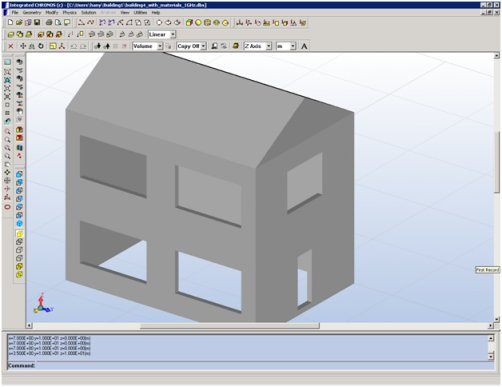Figure 3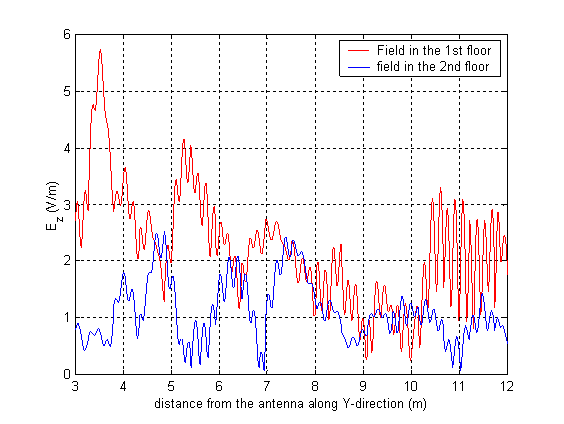Figure 4

Although the above examples show several advantages in using the time domain solver, using a frequency domain solver such as SINGULA has big advantages when solving large electromagnetic problems, especially when the simulation is required at only a few frequencies. Solving large problems that have largest dimension about 100λ is difficult using Boundary Element Method (BEM) / Method of Moments (MoM). For solving such large problems, the Fast Fourier Transfer (FFT) technique was incorporated into the BEM. Figure 5 shows a large Cassegrain parabolic reflector antenna fed by a conical horn radiating a circularly polarized pattern . The focal length and the aperture diameter of the main reflector (paraboloid) are F=1.221m and D=3.8m. The parameters, distance between the foci and the aperture of the hyperbolic subreflector are 1.221 m and 0.3042 m, respectively. Simulations were carried out at a frequency of 10GHz (126.6λ).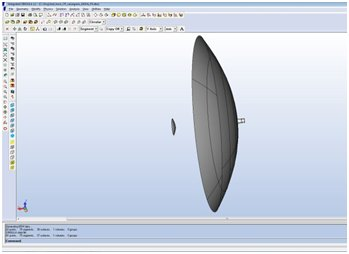Figure 5

Though MoM has many advantages over FEM for the open region electromagnetic field problems, FEM can offer faster solutions for the closed region problems with almost the same solution accuracy as that of MoM. This fact is even more relevant in the determination of the global parameters such as Z, Y, and S matrices of RF circuits. Another application, where FEM can be a better choice, is the determination of the eigenvalues of the microwave resonators of arbitrary shapes. In view of these advantages, FEM solver is also incorporated in our high frequency electromagnetic field solver SINGULA.

As a conclusion, the time domain solver CHRONOS is much faster than the frequency domain solvers especially when the solution is required over a wideband of frequencies, while the frequency domain solver SINGULA gives advantages for solving very large problems when the simulation is required at only a few frequencies or at a single frequency. With the development of CHRONOS, the most powerful innovative techniques of Finite Difference Time Domain, Method of Moments (MoM), and the Finite Element Method (FEM) will be available to the designers in the Integrated’s Software environment .

References:

 Hany E. Abd-El-Raouf, et al., “Design of Double Layered Metamaterial Antenna,” Eucap 2009 3rd European Conference on Antennas and Propagation – 23-27 March 2009 in Berlin, Germany.

 “Simulation of Large Cassegrain Reflector Fed by Point Source in SINGULA”, www.integratedsoft.com, April 2010.

 Tom Judge, “Adaptive BEM and FEM meshing increase confidence in electromagnetic simulation results”, Bodo’s Power System, pp.38-40, December 2009.